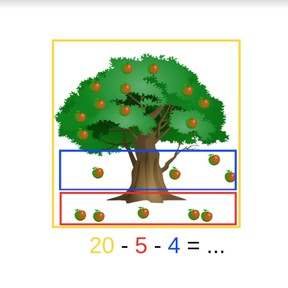Subtraction to 20 with three or more numbers

# Subtraction to 20 with three or more numbers8,000 schools use Gynzy92,000 teachers use Gynzy1,600,000 students use Gynzy

## General

Students learn to subtract numbers to 20 with three or more numbers.

1.OA.C.5
1.OA.C.6

## Relevance

It is important to be able to take away multiple numbers from a total number. In some situations it is required, for example when handing out candies to your friends and you want to know how many you have left.

## Introduction

Start by practicing two subtraction problems with students on the interactive whiteboard. The story problems given have visual support for the subtraction problems. The first subtraction problem has one number to take away, the second has two.

## Development

Start by discussing the importance of being able to subtract with multiple numbers. Next, explain to students how to solve a subtraction problem with multiple numbers (subtrahends) using candies. Tell the students to first look at how many there are in total. This is the first number (minuend) of the subtraction problem. Then decide which number you want to take away first, and subtract that from the first number. Use that difference and subtract the next number from the intermediate difference. Continue until there are no more numbers to take away and you find your difference. Discuss with students that the order of the second, third, etc numbers (subtrahends) does not matter, as long as the first number(minuend) stays in the same place at the start of the subtraction problem. This learning goal is explained in three different methods, visually, in the abstract, and in story form. You can use the blue menu in the bottom right to select the method that is best suited to your classroom needs. With the visual method, use the images to explain how you can subtract with multiple numbers. With the abstract, the subtraction problems are given and you can also see the method of turning your subtraction problem into an addition problem. Finally the steps to solving a story problem are given, with a speaker available to read the stories out loud. For each of the methods, the teacher first explains what the method is, practice subtraction problems are given on the interactive whiteboard, and then problems for the students to try on their own are given.

Check that students are able to subtract three or more numbers up to 20 by asking the following questions:
- Why is it useful to be able to subtract multiple numbers?
- What is your first step when solving a subtraction problem with multiple numbers?
- Why can it be useful to switch the order of the numbers you are subtracting after you've determined the total (first number)?

## Guided practice

Students are first given a subtraction problem with visual support, then an abstract subtraction problem, and finally are given a story problem to solve.

## Closing

To close the lesson, divide the class into groups to play a game with dice. Each group gets 3 dice. At the start of each round, each student starts with 20 points. The numbers they throw (with the three dice) must be subtracted from that student's total of 20 points. A round is complete when each student has thrown the dice and subtracted those numbers from their starting 20 points. The student with the most points wins the round. You can also tell students to play 5 rounds and see who won the most rounds.

## Teaching tips

Students can be supported by doing the subtraction problems with blocks or other manipulatives. Start them on an easy problem like 15 - 1-2-3. And have them move blocks to represent each number that they take away. Remind them that they don't need to subtract in the order they are written, but can choose which one to take away from the total first.

## Instruction materials

3 dice per group
optional: blocks or manipulatives

### The online teaching platform for interactive whiteboards and displays in schools

• Save time building lessons

• Manage the classroom more efficiently

• Increase student engagement